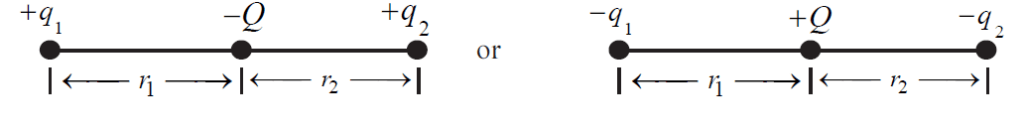# Equilibrium of electric forces on charges on one line & formulas

In this post, we will take a few cases where the Equilibrium of electric forces on charges on one line happens. In every case, there are 3 charges on one line. We will list down the specific equilibrium condition of each such case and the formula of equilibrium for that scenario.

## Scenario 1 (Equilibrium of electric forces)figure 1: 3 charges in one line. the charge in the middle is opposite in polarity to the other 2 charges.

As shown in the figure, all 3 charges are placed on one line.

Now, q1 and q2 are like charges while Q is the unlike charge. (This means, the charge in the middle is opposite in polarity to the other 2 charges)

1 ) When the force on q1 is zero then

q2/Q = (r1 + r2)2/r12

2 ) When the force on q2 is zero then

q1/Q = (r1 + r2)2/r22

3 ) When the force on Q is zero then

q2/q1 = r22/r12

## Scenario 2 (Equilibrium of electric forces)

If all three charges are like charges then the condition for the equilibrium of Q is,

q1/q2 = r12/r22